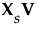• n = number of rows
 • p = number of variables
 • X = n by p matrix of data values
When you select the Wide method, the data are standardized. To standardize a value, subtract its mean and divide by its standard deviation. Denote the n by p matrix of standardized data values by Xs. Then the covariance matrix of the standardized data is the correlation matrix of X and it is given as follows:
Using the singular value decomposition, Xs is written as UDiag(Λ)V’. This representation is used to obtain the eigenvectors and eigenvalues of Xs’Xs. The principal components, or scores, are given by. For additional background information, see Wide Linear Methods and the Singular Value Decomposition in Statistical Details.
Consider the same notation and standardization of X that is described in Wide. The correlation matrix of X is represented by the covariance matrix of Xs:

Help created on 9/19/2017